# Abstract

在本文中，我们提出了 Deep Embedded Clustering(DEC)，一种使用深度神经网络同时学习 feature representations 和 cluster assignments 的方法。DEC学习从数据空间到低维特征空间的映射，并在其中迭代地优化聚类目标。

# 1. Introduction

What is Clustering???

Some questions for Clustering in unsupervised methods:

• What defifines a cluster?
• What is the right distance metric?
• How to effificiently group instances into clusters?
• How to validate clusters?
DEC的目的：本文希望找到一种方案，可以联合解决特征空间学习与聚类关系判别
本文定义了一个从 data space $X$ 到 lower-dimensional feature space $Z$ 的参数化 non-linear mapping ，在其中优化了一个聚类目标。之前的工作主要在数据空间或浅层线性嵌入空间上操作，本文通过在聚类目标上的反向传播使用随机梯度下降(SGD)来学习映射，这是由深度神经网络参数化的。本文将这种聚类算法称为 Deep Embedded Clustering ，或DEC。

本文贡献：

1. joint optimization of deep embedding and clustering;
2. a novel iterative refifinement via soft assignment;
3. state-of-the-art clustering results in terms of clustering accuracy and speed.

# 2. Related work

聚类在机器学习的  feature selection、distance functions、grouping methods 以及 cluster validation 方面得到了广泛研究。

流行的聚类方法的一个分支是 kmeans 和高斯混合模型(GMM)。这些方法速度快，可适用于范围广泛的问题。然而，它们的距离度量仅限于原始数据空间，当输入维数较高时，它们往往会无效。

Spectral clustering 及其变体最近得到了受欢迎。它们允许更灵活的距离度量，并且通常比 kmeans 表现得更好。Yang等人已经探索了 spectral clustering 和 embedding 的结合。 Tian 等人提出了一种基于 spectral clustering 的算法，但用深度自编码器代替特征值分解，提高了性能，但进一步增加了内存消耗。

Spectral clustering algorithms 需要计算全图的  Laplacian matrix ，因此在数据点的数量上具有二次或超二次复杂度。

最小化 data distribution 和 embedded distribution 之间的 KL   差异。

我们从参数化的  $t-SNE$  中获得灵感。我们定义了一个 centroid-based  的概率分布，并最小化其  $KL$  散度到辅助目标分布，以同时改进聚类分配和特征表示，而不是最小化  $KL$  散度以产生辅助目标分布，从而产生忠实于原始数据空间中距离的嵌入。基于质心的方法也有将复杂度降低到  $O(nk)$  的好处，其中  $k$  是质心的数量。

# 3. Deep embedded clustering

Consider the problem of clustering a set of  $n$  points  $\left\{x_{i} \in\right. X\}_{i=1}^{n}$ into  $k$  clusters, each represented by a centroid  $\mu_{j}$, $j=1, \ldots, k$. Instead of clustering directly in the data space  $X$ , we propose to first transform the data with a nonlinear mapping  $f_{\theta}: X \rightarrow Z$ , where  $\theta$  are learnable parameters and  $Z$ is the latent feature space. The dimensionality of  $Z$  is typically much smaller than  $X$  in order to avoid the "curse of dimensionality" (Bellman, 1961). To parametrize  $f_{\theta}$ , deep neural networks (DNNs) are a natural choice due to their theoretical function approximation properties (Hornik, 1991) and their demonstrated feature learning capabilities (Bengio et al., 2013).
The proposed algorithm (DEC) clusters data by simultaneously learning a set of  $k$  cluster centers  $\left\{\mu_{j} \in Z\right\}_{j=1}^{k}$  in the feature space  $Z$  and the parameters  $\theta$  of the DNN that maps data points into  $Z$ . DEC has two phases: (1) parameter initialization with a deep autoencoder (Vincent et al., 2010) and (2) parameter optimization (i.e., clustering), where we iterate between computing an auxiliary target distribution and minimizing the Kullback-Leibler (KL) divergence to it. We start by describing phase (2) parameter optimization  clustering, given an initial estimate of  $\theta$  and  $\left\{\mu_{j}\right\}_{j=1}^{k}$.

## 3.1. Clustering with KL divergence

给定非线性映射  $f_{\theta}$  和初始聚类质心 $\left\{\mu_{j}\right\}_{j=1}^{k}$  的初始估计，我们建议改进聚类使用在两个步骤之间交替的无监督算法。 第一步，我们计算  embedded points  和  cluster centroids  之间的  soft assignment 。 第二步，我们更新深度映射  $f_{\theta}$  并通过使用辅助目标分布从当前的高置信度分配中学习来细化集群质心。 重复此过程，直到满足收敛标准。

### 3.1.1. Soft assignment

我们使用 Student’s t-distribution 作为核来衡量 embedded point  $z_i$  和质心  $\mu_{j}$  之间的相似性：

$q_{i j}=\frac{\left(1+\left\|z_{i}-\mu_{j}\right\|^{2} / \alpha\right)^{-\frac{\alpha+1}{2}}}{\sum_{j^{\prime}}\left(1+\left\|z_{i}-\mu_{j^{\prime}}\right\|^{2} / \alpha\right)^{-\frac{\alpha+1}{2}}} \quad \quad\quad\quad (1)$

其中：$z_{i}=f_{\theta}\left(x_{i}\right) \in Z$  是嵌入后的  $x_{i} \in X$  ，$\alpha$  是  Student's  t  distribution 的自由度，$q_{i j}$  可以解释为将样本 $i$ 分配给聚类 $j$ 的概率（即软分配）。由于我们不能在无监督设置下的验证集上交叉验证  $\alpha$，并且学习它是多余的，我们让  $α=1$  用于所有实验。

### 3.1.2. KL Divergence minimization

我们建议在  auxiliary target distribution 的帮助下，通过学习  high confidence assignments 来迭代地细化  clusters 。具体来说，我们的模型是通过匹配软分配到目标分布来训练的。为此，我们将我们的目标定义为软分配  $q_i$  和辅助分布  $p_i$  之间的KL散度损失如下：

$L=\mathrm{KL}(P \| Q)=\sum \limits_{i} \sum \limits_{j} p_{i j} \log \frac{p_{i j}}{q_{i j}} \quad \quad\quad\quad (2)$

目标分布  $P$  的选择是  DEC  的性能的关键。一种简单的方法是将超过置信阈值的数据点的每个  $p_i$  设置为一个  $delta$  分布（到最近的质心），而忽略其余的。然而，由于  $q_i$  是软任务，所以使用较软的概率目标更自然和灵活。

具体来说，我们希望我们的  target distribution  具有以下特性：

1. strengthen predictions (i.e., improve cluster purity).
2. put more emphasis on data points assigned with high confidence.
3. normalize loss contribution  of each centroid to prevent large clusters from distorting the hidden feature space.

在我们的实验中，我们计算  $p_i$，首先将  $q_i$  提高到二次幂，然后按每个簇的频率归一化：

${\large p_{i j}=\frac{q_{i j}^{2} / f_{j}}{\sum_{j^{\prime}} q_{i j^{\prime}}^{2} / f_{j^{\prime}}}} \quad \quad\quad\quad (3)$

其中  $f_{j}=\sum \limits _{i} q_{i j}$   是 soft cluster frequencies 。关于L和P的经验性质的讨论，请参考第5.1节。

### 3.1.3. Optimization

我们利用具有动量的随机梯度下降(SGD)联合优化了聚类中心  $\left\{\mu_{j}\right\}$  和DNN参数  $\theta$。$L$ 对每个数据点  $z_i$  和每个聚类质心  $\mu_{j}$  的特征空间嵌入的梯度计算为：

$\frac{\partial L}{\partial z_{i}}=\frac{\alpha+1}{\alpha} \sum \limits _{j}\left(1+\frac{\left\|z_{i}-\mu_{j}\right\|^{2}}{\alpha}\right)^{-1}\quad \quad\quad\quad (4)$

$\frac{\partial L}{\partial \mu_{j}}=-\frac{\alpha+1}{\alpha} \sum \limits _{i}\left(1+\frac{\left\|z_{i}-\mu_{j}\right\|^{2}}{\alpha}\right)^{-1}\times\left(p_{i j}-q_{i j}\right)\left(z_{i}-\mu_{j}\right)\quad \quad\quad\quad (5)$

梯度  $\partial L / \partial z_{i}$  然后被传递给DNN，并用于标准的反向传播来计算  DNN  的参数梯度  $\partial L / \partial \theta$。为了发现集群分配，当连续两次迭代之间改变集群分配的点小于  $tol \%$  时，我们停止我们的过程。

## 3.2. Parameter initialization

到目前为止，我们已经讨论了  DEC  如何处理  DNN  参数$θ$  和簇质心  ${µ_j}$  的初始估计。现在我们将讨论参数和质心是如何初始化的。

我们使用堆叠自动编码器(SAE)初始化DEC，因为最近的研究表明，它们在真实数据集上始终产生具有语义意义和分离良好的表示。因此，SAE学习到的无监督表示自然地促进了使用DEC进行聚类表示的学习。

我们一层一层地初始化SAE网络，每一层都是一个去噪自动编码器，在随机损坏后重建前一层的输出。去噪自动编码器是一种两层神经网络，其定义为：

$\tilde{x} \sim \operatorname{Dropout}(x) \quad \quad\quad\quad (6)$

$h=g_{1}\left(W_{1} \tilde{x}+b_{1}\right) \quad \quad\quad\quad (7)$

$\tilde{h} \sim \operatorname{Dropout}(h) \quad \quad\quad\quad (8)$

$y=g_{2}\left(W_{2} \tilde{h}+b_{2}\right)\quad \quad\quad\quad (9)$

其中，$\operatorname{Dropout}(\cdot)$  是一个随机映射，它将其输入维度的一部分随机设置为0，$g_{1}$  and  $g_{2}$ 分别为编码层和解码层的激活函数，$\theta=\left\{W_{1}, b_{1}, W_{2}, b_{2}\right\}$  为模型参数。训练是通过最小化最小二乘损失  $\|x-y\|_{2}^{2}$  来完成的。在训练了一层后，我们使用它的输出有输入来训练下一层。我们在所有编码器/解码器对中使用校正线性单元(ReLUs)，除了第一对的  $g_{2}$ （它需要重建可能有正负值的输入数据，如零均值图像）和最后对的  $g_{1}$  (因此最终数据嵌入保留完整信息)。

经过  greedy layer-wise training  后，我们将所有编码器层和所有解码器层，按反向层训练顺序连接起来，形成一个深度自动编码器，然后对其进行微调，使其最小化重构损失。最终的结果是一个中间有一个瓶颈编码层的多层深度自动编码器。然后我们丢弃解码器层，并使用编码器层作为数据空间和特征空间之间的初始映射，如 Fig. 1 所示。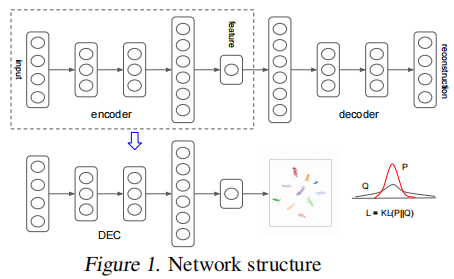为了初始化聚类中心，我们将数据通过初始化的DNN传递，得到嵌入的数据点，然后在特征空间  $Z$  中执行标准的  $k-means$  聚类，得到  $k$  个初始质心  $\left\{\mu_{j}\right\}_{j=1}^{k}$。

# 4. Experiments

## 4.1. Datasets

我们在一个文本数据集和两个图像数据集上评估了所提出的方法(DEC)，并将其与其他算法包括  $k-means$、$LDGMI$ 和  $SEC$  进行比较。$LDGMI$  和$SEC$  是基于  spectral clustering  的算法，它们使用拉普拉斯矩阵和各种变换来提高聚类性能。

• MNIST：MNIST数据集由280000像素大小的70000个手写数字组成。这些数字居中并进行尺寸归一化(LeCun等，1998)。
• STL-10: 96 x 96彩色图像的数据集。有10个类别，每个类别有1300个样本。它还包含100000张相同分辨率的无标签图像(Coates等，2011)。训练自动编码器时，我们还使用了无标签的集合。与Doersch等人(2012)相似，我们将HOG特征和8×8色图连接起来，用作所有算法的输入。
• REUTERS: REUTERS包含大约810000个以类别树标签的英语新闻报道(Lewis等，2004)。我们使用了四个根类别：公司/工业，政府/社会，市场和经济学作为标签，并进一步修剪了由多个根类别标签的所有文档以得到685071个文章。然后，我们根据2000个最常见的词干计算tf-idf特征。由于某些算法无法扩展到整个Reuters数据集，因此我们还抽样了10000个样本的随机子集，我们将其称为REUTERS-10k，以进行比较。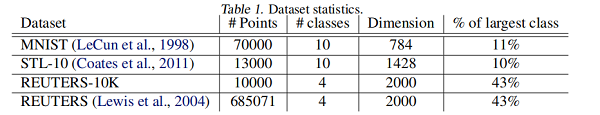## 4.2. Evaluation Metric

我们使用标准的无监督评估  metric  和   protocol  来与其他算法进行评估和比较。对于所有的算法，我们将聚类的数量设置为 ground-truth  类别的数量，并以无监督的聚类精度(ACC)来评估性能：

$A C C=\max _{m} \frac{\sum_{i=1}^{n} 1\left\{l_{i}=m\left(c_{i}\right)\right\}}{n}\quad \quad\quad\quad (10)$

其中 $l_{i}$  是ground-truth label ，$c_{i}$ 是该算法产生的聚类分配，$m$ 是将  $c_{i}$ 进行聚类映射。

## 4.3. Implementation

实验参数补充，略........

## 4.4. Experiment results

我们定量和定性地评估了我们的算法的性能。在 Table 2 中，我们报告了每种算法的最佳性能，超过9个超参数设置。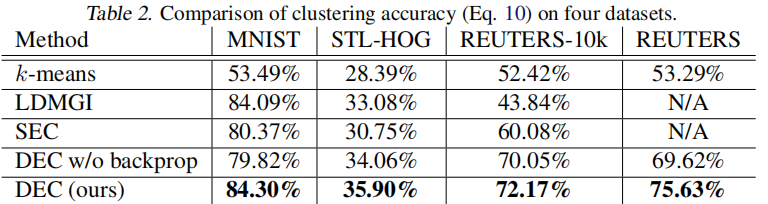请注意，DEC的性能优于所有其他方法，有时会有很大的优势。为了证明端到端训练的有效性，我们还展示了在聚类过程中冻结非线性映射  $f_θ$  的结果。

为了研究超参数的影响，我们绘制了每种方法在所有 $9$ 种设置下的准确性（ Fig.2）。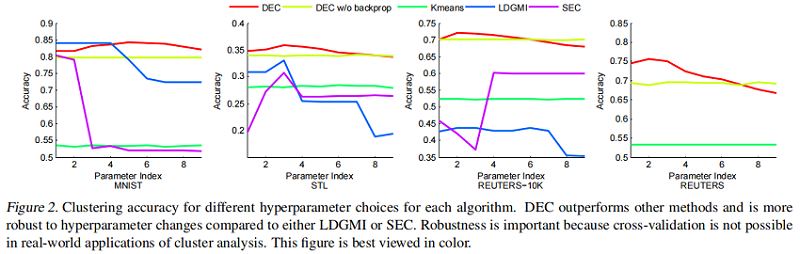我们观察到，与  LDGMI  和  SEC  相比，DEC  在超参数范围内更为一致。对于  DEC，超参数  $λ=40$  在所有数据集上都给出了接近最优的性能，而对于其他算法，最优超参数变化很大。此外，DEC可以用GPU加速处理整个  REUTERS  数据集，而第二好的算法LDGMI和SEC则需要数月的计算时间和 TB 的内存。事实上，我们不能在完整的  REUTERS  数据集上运行这些方法，并在  Table 2  中报告N/A(这些方法的GPU适应不是重要的)。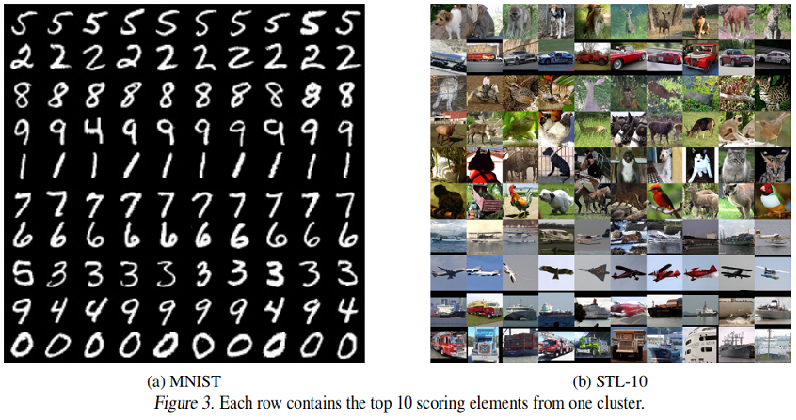在  Fig. 3  中，我们显示了  MNIST  和  STL  中每个聚类中10张得分最高的图像。每一行对应一个  cluster，图像根据它们到  cluster center  的距离从左到右进行排序。我们观察到，对于  MNIST，DEC  的  cluster assignment  很好地对应自然集群，除了混淆4和9，而对于STL，DEC对飞机、卡车和汽车大多是正确的，但在动物类别时，部分注意力花在姿势上而不是类别上。

# 5. Discussion

## 5.1. Assumptions and Objective

DEC的基本假设是，初始分类器的高置信度预测大部分是正确的。为了验证该假设对我们的任务是否成立，以及我们对  $P$  的选择是否具有所需的属性，我们针对每个嵌入点  $\left|\partial L / \partial z_{i}\right|$  ，对其软分配  $q_{ij}$  ，绘制了  $L$  的梯度大小到随机选择的  MNIST  聚类  $j$(Fig4)。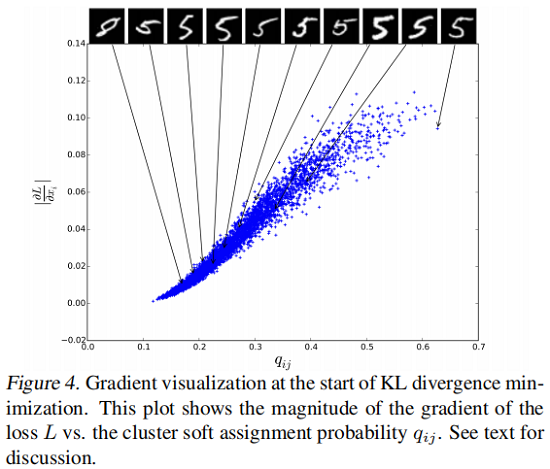我们观察到靠近聚类中心(较大  $q_{ij}$  )的点对梯度的贡献更大。我们还显示了按  $q_{ij}$   排序的每个10个百分位数的10个数据点的原始图像。如相似度更高的实例“ 5”。随着置信度的降低，实例变得更加模棱两可，最终变成错误标签的 ”8”，表明我们的假设是正确的。

## 5.2. Contribution of Iterative Optimization

在  Fig.5  中，我们可视化了训练过程中  MNIST  随机子集的嵌入式表示的进度。为了可视化，我们将  t-SNE应用于嵌入点  $z_i$。显然，聚类之间的隔离度越来越高。 Fig.5(f)  显示了在SGD epoch  相对应的精度如何提高。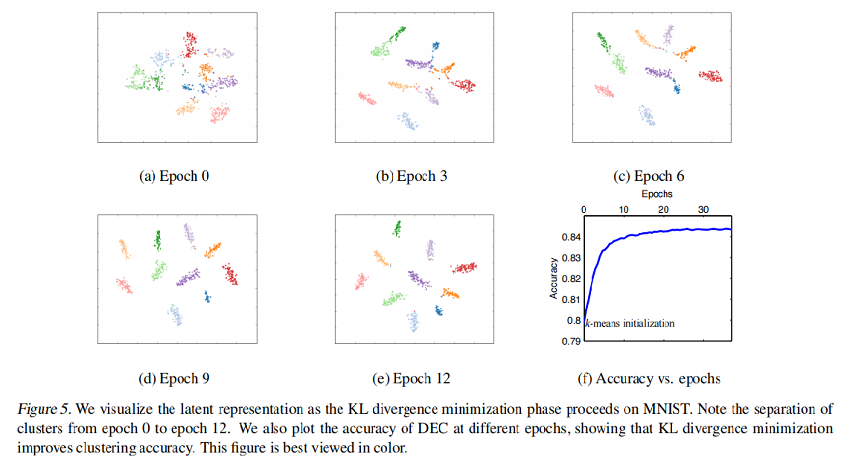## 5.3. Contribution of Autoencoder Initialization

为了更好地理解每个组件的贡献，我们在 Table 3 中展示了所有具有自编码器特征的算法的性能。我们观察到，SEC  和  LDMGI  的表现并没有随  autoencoder feature  而显著变化，而  $k-means$  有所改善，但仍低于  $DEC$  。这证明了采用提议的  $KL$  散度目标进行深度嵌入的能力以及微调的好处。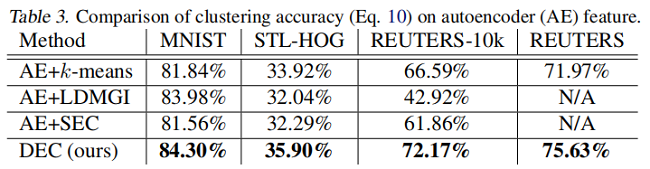## 5.4. Performance on Imbalanced Data

为了研究不平衡数据的影响，我们对具有不同保留率的  MNIST  子集进行了采样。对于最小保留率  $r_{\min }$，类别  $0$  的数据点将以  $r_{\min }$的概率保留，类别  $9$  将以  $1$  的概率保留，而其他类别的数据点之间则保持线性关系。结果，最大的簇将是最小簇的  $1 / r_{\min }$  倍。从  Table 4  中我们可以看到  DEC  对于簇大小的变化具有相当强的鲁棒性。我们还观察到，在自动编码器和  k-means  初始化（显示为AE+k-means）之后，KL散度最小化(DEC)不断提高了聚类精度。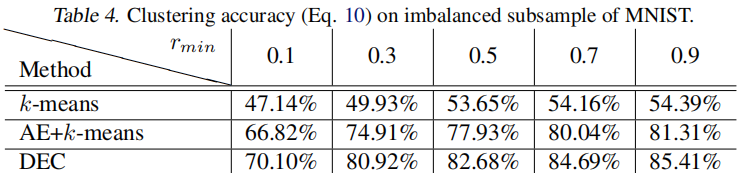## 5.5. Number of Clusters

到目前为止，我们已经假定给出自然簇的数量是为了简化算法之间的比较。但是，实际上，此数量通常是未知的。因此，需要一种确定最佳簇数的方法。为此，我们定义两个度量：

• 标准度量，归一化互信息(NMI)，用于评估具有不同聚类数量的聚类结果：

$N M I(l, c)=\frac{I(l, c)}{\frac{1}{2}[H(l)+H(c)]}$

其中  $I$  是互信息度量，$H$ 是熵。

• 泛化性(G)定义为训练损失与验证损失之间的比率：

$G=\frac{L_{\text {train }}}{L_{\text {validation }}}$

当训练损失低于验证损失时，$G$  很小，这表明高度过拟合。

Fig. 6  显示了当簇数从  $9$ 增加到  $10$  时，泛化性急剧下降，这表明  $9$ 是最优的簇数。我们确实观察到NMI得分最高为  $9$，这表明泛化性是选择簇数的良好指标。 NMI最高是  $9$，而不是  $10$，因为  $9$  和  $4$  在文字上相似，DEC认为它们应该组成一个聚类。这与我们在  Fig. 3  中的定性结果非常吻合。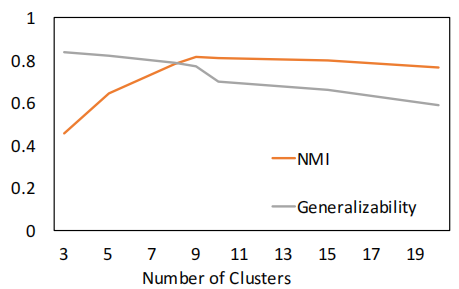# 6. Conclusion

本文提出了深度嵌入式聚类，即DEC，一种在联合优化的特征空间中聚集一组数据点的算法。DEC的工作原理是迭代优化基于KL散度的聚类目标和自训练目标分布。我们的方法可以看作是半监督自我训练的无监督扩展。我们的框架提供了一种方法来学习专门的表示，而没有基本聚类成员标签。

实证研究证明了我们所提出的算法的有效性。DEC提供了更好的性能和鲁棒性，这在无监督任务中尤其重要，因为交叉验证是不可能的。DEC还具有数据点数量的线性复杂性的优点，这使得它可以扩展到大型数据集。

『总结不易，加个关注呗！』posted @ 2022-01-12 20:56  Learner-  阅读(450)  评论(0编辑  收藏  举报